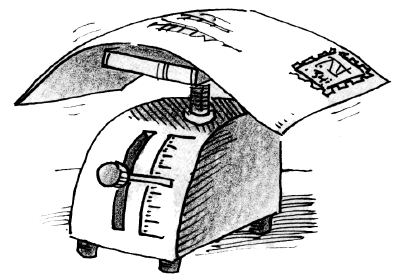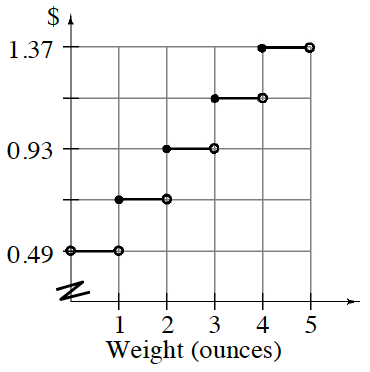Home > APCALC > Chapter 1 > Lesson 1.2.1 > Problem1-22

1-22.

In order to mail a letter in the United States, postage must be paid based on the weight of the letter. Although rates are tied to the number of ounces, the U.S. Post Office does not allow for payments of partial ounces.A graph showing the postage rates for letters weighing fewer than $5$ ounces for the year 2015 is shown at right. This is another example of a step function1. How much would you pay for a letter weighing $2.9$ ounces? For $3$ ounces? For $3.1$ ounces?

This piecewise function has five steps (or pieces). Look closely at the Boundary Points between pieces: $x = 1$ oz, $x = 2$ oz, $x = 3$ oz, $x = 4$ oz, etc. Will you pay a higher or lower rate if your item weighed exactly any of those amounts?

2. Write a piecewise-defined function that determines the postage rates for letters weighing between $0$ and $5$ ounces. Let $x$ represent the weight in ounces, and $y$ represent cost in dollars.

Notice that this piecewise function consists of five horizontal pieces. Recall that a horizontal line is of the form $y = k$, where $k$ is a constant. Also, consider the boundary points: will $≤$ or $≥$ be appropriate?

$\begin{equation} y=\left\{ \begin{array}{@{}ll@{}} 0.44 & \text{for}\ 0 \leq x \leq 1 \\ 0.61 & \text{for}\ 1